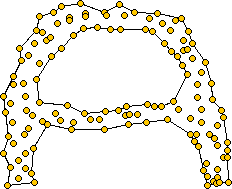# 计算Alpha Shape（与K-Means聚类）

### 谢益辉 / 2009-02-20

set.seed(1234)
par(pch = 20)
dat = iris[, 1:2]
n = nrow(dat)
for (k in 2:30) {
ch = integer()
cl = kmeans(dat, k, 50)\$cluster
plot(dat, main = paste("k =", k))
for (i in unique(cl)) {
idx = chull(tmp <- dat[cl == i, ])
ch = c(ch, as.integer(rownames(tmp[idx, ])))
polygon(tmp[idx, ], border = NA, col = rgb(0, 0, 0, 0.2))
}
plot(dat, main = paste("Polygon shape when k =", k))
polygon(dat[ch, ], col = rgb(0, 0, 0, 0.2))  # need to be ordered
}